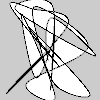# Py5Shape.curve_vertices()#

Create a collection of curve vertices.

## Examples#import numpy as np

def setup():
py5.size(100, 100, py5.P2D)
random_curve_vertices = 100 * np.random.rand(25, 2)
s = py5.create_shape()
with s.begin_shape():
s.vertex(py5.width / 2, py5.height / 2)
s.curve_vertices(random_curve_vertices)
py5.shape(s)


## Description#

Create a collection of curve vertices. The purpose of this method is to provide an alternative to repeatedly calling Py5Shape.curve_vertex() in a loop. For a large number of curve vertices, the performance of curve_vertices() will be much faster.

The coordinates parameter should be a numpy array with one row for each curve vertex. There should be two or three columns for 2D or 3D points, respectively.

Drawing 2D curves requires using the P2D renderer and drawing 3D curves requires using the P3D renderer. When drawing directly with Py5Shape objects, curves do not work at all using the default renderer.

This method can only be used within a Py5Shape.begin_shape() and Py5Shape.end_shape() pair.

## Signatures#

curve_vertices(
coordinates: npt.NDArray[np.floating],  # 2D array of curve vertex coordinates with 2 or 3 columns for 2D or 3D points, respectively
/,
) -> None


Updated on June 26, 2023 01:48:37am UTC